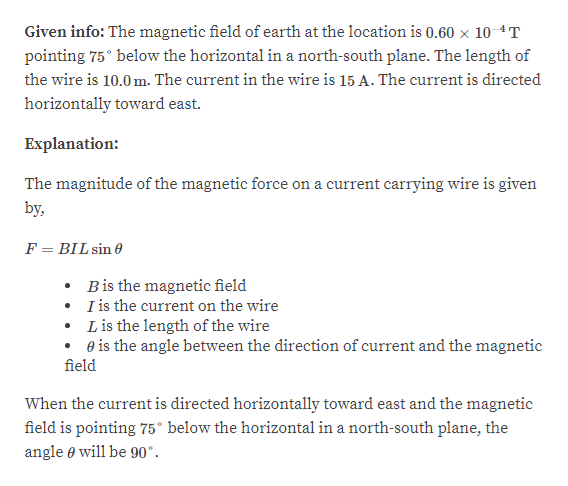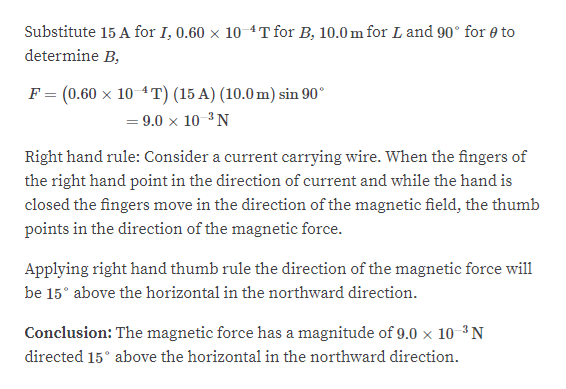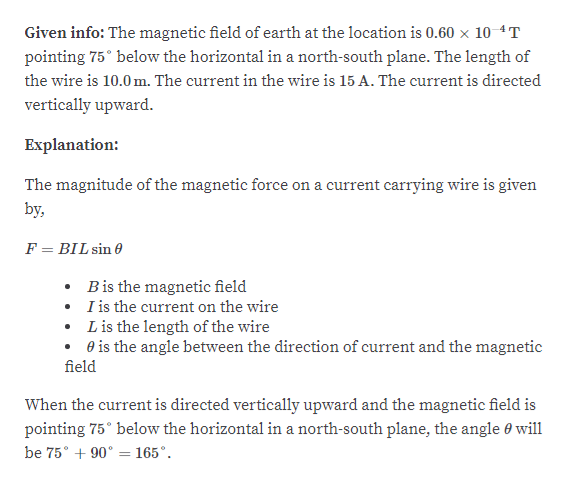# At a certain location, Earth has a magnetic field of 0.60 x10-4 T, pointing 75° below the horizontal in a north–southplane. A 10.0 - m - long straight wire carries a 15 - A current.(a) If the current is directed horizontally toward the east,what are the magnitude and direction of the magnetic forceon the wire? (b) What are the magnitude and direction of theforce if the current is directed vertically upward?

Question
41 views

At a certain location, Earth has a magnetic field of 0.60 x
10-4 T, pointing 75° below the horizontal in a north–south
plane. A 10.0 - m - long straight wire carries a 15 - A current.
(a) If the current is directed horizontally toward the east,
what are the magnitude and direction of the magnetic force
on the wire? (b) What are the magnitude and direction of the
force if the current is directed vertically upward?

check_circle

Step 1

Part a:help_outlineImage TranscriptioncloseGiven info: The magnetic field of earth at the location is 0.60 x 10 4T pointing 75° below the horizontal in a north-south plane. The length of the wire is 10.0 m. The current in the wire is 15 A. The current is directed horizontally toward east. Explanation: The magnitude of the magnetic force on a current carrying wire is given by, F = BIL sin 0 Bis the magnetic field I is the current on the wire L is the length of the wire e is the angle between the direction of current and the magnetic field When the current is directed horizontally toward east and the magnetic field is pointing 75° below the horizontal in a north-south plane, the angle e will be 90°. fullscreen
Step 2help_outlineImage TranscriptioncloseSubstitute 15 A for I, 0.60 x 10 4T for B, 10.0 m for L and 90° for e to determine B, F = (0.60 x 10 4T) (15 A) (10.0 m) sin 90° = 9.0 x 10 ³ N Right hand rule: Consider a current carrying wire. When the fingers of the right hand point in the direction of current and while the hand is closed the fingers move in the direction of the magnetic field, the thumb points in the direction of the magnetic force. Applying right hand thumb rule the direction of the magnetic force will be 15° above the horizontal in the northward direction. Conclusion: The magnetic force has a magnitude of 9.0 × 10 ³N directed 15° above the horizontal in the northward direction. fullscreen
Step 3

Part b:

...help_outlineImage TranscriptioncloseGiven info: The magnetic field of earth at the location is 0.60 x 10 4T pointing 75° below the horizontal in a north-south plane. The length of the wire is 10.0 m. The current in the wire is 15 A. The current is directed vertically upward. Explanation: The magnitude of the magnetic force on a current carrying wire is given by, F = BIL sin 0 Bis the magnetic field I is the current on the wire • L is the length of the wire • e is the angle between the direction of current and the magnetic field When the current is directed vertically upward and the magnetic field is pointing 75° below the horizontal in a north-south plane, the angle 0 will be 75° + 90° = 165°. fullscreen

### Want to see the full answer?

See Solution

#### Want to see this answer and more?

Solutions are written by subject experts who are available 24/7. Questions are typically answered within 1 hour.*

See Solution
*Response times may vary by subject and question.
Tagged in

### Electromagnetic Induction